Abstract

The focus of this discussion is to explore the benefits of 4-valve head design versus a conventional 2-valve head.  The specific area of focus is airflow capacity of the intake valves and it’s effect on engine performance.

Introduction

The ratio of intake air velocity to the speed of sound in the intake gases is referred to as the Mach Index (Z).  Airflow studies have shown that as the Mach Index values exceed .6, volumetric efficiency (Ve) falls rapidly.  The Mach Index number is defined as: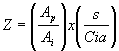where

Z = Mach Index

Ap = Area of the piston

Ai = Intake valve area x # of intake valves

D = Intake Valve diameter

s = Mean Piston Speed

a = Velocity of Sound in intake gases

Ci = Mean Valve Flow Co-efficient

If we can calculate the maximum airflow through a valve (or valves) before we exceed Z .6, we can then calculate how much potential power a given engine design has.

For this discussion, I’ll use the geometry for the 1981 Euro 635CSi engine for our 2-valve engine.  It’s bore size and piston area is virtually identical to the 1985 Euro M5 engine from which we’ll utilize the 4-valve geometry.  The various specifications are as follows:

Bore – 93.36mm

Intake valve – 46mm x 1

Intake Valve – 37mm x 2

Maximum Mean Piston Speeds

Rearranging our Mach Index formula, we can solve it to find maximum ‘s’ without exceeding Z .6.  Because this is a theoretical example designed to show the difference in and effect of airflow between 2 and 4-valve head designs, a number of experience-derived numbers will be utilized.  Hence, we will assume the following:

a = 1200 ft/min or 366m/sec

Ci­ = .3 (though real-world values vary from <.3 to >.4)

s = Z /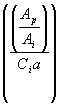= .6 /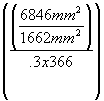= .6 / .0375 = 16m/sec

Maximum Mean Piston Speed for our 2-valve engine is 16m/sec or 3150ft/min.

Calculating the formula with the 4-valve head Ai we obtain the following:

s = Z /= .6 /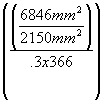= .6 / .0290 = 20.7m/sec

Maximum Mean Piston Speed for our 4-valve engine is 20.7m/sec or 4075ft/min, an increase of 29%.

Rated Power

Rated Power (HP at a given engine speed) can be calculated as follows:

P =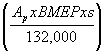where

P = Horsepower

Ap = Area of the piston

BMEP = Break Mean Effective Pressure in psi

s = Mean Piston Speed

BMEP is Indicated Mean Effective Pressure – Friction Mean Effective Pressure – Pumping Mean Effective Pressure of a given engine.  It takes into account the energy consumed by the engine itself in frictional losses and pumping work (pushing exhaust gases etc.)  Once again, as this is a theoretical exercise concerned only with airflow, the BMEP value will be based on values gleaned from real-world data collection.

P =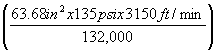= 205hp

P =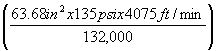= 265hp

For argument’s sake, let’s check out what happens when we hotrod our 2-valve head with a larger 47mm valve.

Max s rises from 3150 to 3287ft/min, and power from 205 to 214hp.

Conclusion

While the preceding discussion was based strictly on mathematical formulas, these formulas have evolved over time based on hundreds of thousands if not millions of hours of real world testing of the internal combustion engine.  The examples clearly show a horsepower increase in the order of 29% as predicted by the 29% increase in airflow.